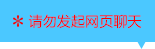| | |

||
APP端下载AndroidiPhone
￥158

|
2276人点赞
149085人已学习
|

3天无理由退款

3天无理由退款：退款将以超级币形式退至您的超级课堂学习账户，便于您重新选购其他课程。恶意退款将被冻结账号。

• 1、正弦就是对边比斜边，余弦就是邻边比斜边，正切就是对边比邻边
2、 三角函数就是一种以锐角为自变量，以比值为函数值的函数。三角函数值就是一个比例
3、 三角函数值的求法，知道直角三角形中的任意两边，或者任意两边的比例，或者任意一个三角函数值，都能利用勾股定理，把每种三角函数值都求出来
4、 给定图形中求某个角的三角函数值。必须在直角三角形里求，如果所求的角并不在直角三角形里，可以采取构造法或角度替换法来解决
• 1、记住三种特殊角的三角函数值
2、 角度和三角函数值的“一一对应”关系。在锐角范围内，一个角对一个值，一个值也对一个角。所以可以通过特殊角得到特殊值，也可以通过特殊值得到特殊角
3、 利用构造法，求15度和75度的三角函数值
• 1、包括平方关系：$sin^{2}\alpha +cos^{2}\alpha =1$和商数关系： $tan\alpha =\frac{sin\alpha }{cos\alpha }$
2、 利用平方关系，可以实现正弦与余弦的互化，关于解题蕴藏着一大技巧，那就是正弦、余弦的和、差、积，知一求二
3、 利用商数关系，可以实现“弦化切”
4、 对已知式子进行变形，采用整体思想代入求解。含有三角函数的式子，对1要灵活处理，经常利用平方关系，化身为$sin^{2}\alpha +cos^{2}\alpha$
• 1、要记住三个基本公式$sin(90^{\circ}-\alpha )=cos\alpha ^{2}$ $cos(90^{\circ}-\alpha )=sin\alpha ^{3}$
2、 $tan(90^{\circ}-\alpha )=\frac{1}{tan\alpha }$
3、 和平方关系联系在一起起又推出了两个新的公式$sin^{2}(90^{\circ}-\alpha )+sin^{2}\alpha =1cos^{2}(90^{\circ}-\alpha )+cos^{2}\alpha =1$，记为互余两角正弦或余弦的平方和都是$1$
4、 通过几道例题向你展示了分组思想的巧妙，你要对和为$90^{\circ}$的一对角度特别敏感，顺利的完成配对
• 1、有界性确定了三角函数值整体的范围：$0＜ sin\alpha ＜ 1,0＜ cos\alpha ＜ 1,tan\alpha ＞0$。我们通过它来验证所求值是否可能成为三角函数值
2、 增减性确定了在锐角范围内，$sin\alpha$和$tan\alpha$会随着$\alpha$的增大而增大，而$cos\alpha$会随着$\alpha$的增大而减小
3、 应用有三点：应用(1)：同一种三角函数不同角之间的大小比较。对$sin\alpha$和 $tan\alpha$角越大则值越大，值越大则角越大；对于$cos\alpha$，角越大则值越小，值越大则角越小
4、 应用(2)：同一个角度，不同三角函数之间的大小比较（1）比较$sin\alpha$和$cos\alpha$：当$\alpha ＜45^{\circ}$时，$sin\alpha＜ cos\alpha$；当$\alpha＞ 45^{\circ}$时$sin\alpha ＞cos\alpha$；当$\alpha= 45^{\circ}$时，$sin\alpha =cos\alpha$。（2）比较$sin\alpha$和$tan\alpha$：在锐角范围内$sin\alpha$始终小于$tan\alpha$。
5、 应用(3)：确定范围，根据角的范围确定三角函数值的范围，或根据三角函数值的范围确定角的范围
• 1、解直角三角形的概念，“在直角三角形中，由已知元素求未知元素的过程”。它遵循知二求三的原则，包含两种情况：已知两边或已知一边一角。由边求边用勾股，由角求角用互余，边角互求用三角函数。这就是解直角三角形的依据
2、 三组关系，一组是$a=csinA$和$b=csinB$，简记为“直角边等于斜边乘以对角的正弦”。第二组是$a=ccosB$和$b=ccosA$，简记为“直角边等于斜边乘以邻角的余弦”。第三组是$a=btanA$和$b=atanB$，简记为“直角边等于另一直角边乘以对角的正切”
• 1、主要认识了三角函数的两种题型，一种是三角形嵌套模型，在利用三角函数求复杂图形的边长时，要注意将条件凑到一个直角三角形中
2、 第二种是在圆上解直角三角形，要学会利用直径构造直角三角形，利用圆周角定理进行角的转化
• 1、要确定一个一般三角形的形状，至少要知道三个元素，而且至少要知道一条边的长度
2、 常见题型有两大类：已知两角一边和已知两边一角。其中两角一边可分为两角+对边和两角+夹边，两边一角可分为两边+夹角和两边+对角
3、 “作高”的技巧，过未知角的顶角作高，在作高时要保留已知角。而且除了已知两边+夹角的情况，尽量保留已知边，充分利用所给的边、角条件。唯一需要注意的是两边+对角的题型，有两种情况，要分类讨论。
4、 一道较难的题目，提醒我们注意画图，当无法直接求出边长时，可以选择尝试用勾股定理列方程
• 1、三角形的面积公式$S=\frac{1}{2absin\alpha }$，即任意两边的乘积乘以它们夹角的正弦除以$2$。当$\angle C$为直角时，$sinC$取$1$；当$\angle C$为钝角时，$C$取$\angle C$的补角。除了计算面积，在证明题当中，面积公式也有妙用
2、 平行四边形的面积公式$S=absin\alpha$。即任意两边的乘积乘以它们夹角的正弦。若$\alpha$是直角，则$sin\alpha$取$1$；若$\alpha$是钝角，则$sin\alpha$取$sin(180^{\circ}-\alpha )$
3、 四边形的面积公式为$S=\frac{1}{2absin\theta }$。即四边形的面积等于对角线乘积乘以它们夹角的正弦除以$2$。当对角线垂直时，$sin\theta$取$1$
4、 几个面积公式，都有正弦函数。变身华丽后的公式，让我们求面积，用面积法，都变得更加自如
• 1、在测量时，视线与水平线所成的角中，视线在水平线上方的叫仰角，视线在水平线下方的叫俯角
2、 关于解决实际测量应用题，超级课堂总结了两种基本图形，与各自旋转$90^{\circ}$后的变形
3、 如果当测量点不在地面时，不要忘记把人或仪器的高度加上去
4、 一种基本图形的变相应用，通过辅助线来构造基本图形
• 1、坡面的铅直高度$h$和水平宽度l的比叫做坡面的坡度，也叫坡比，一般用$i$来表示，坡面与水平面的夹角$\alpha$叫做坡角。坡度是坡角的正切，即$i=tan\alpha$
2、 坡度也可以用任意两点垂直距离与水平距离的比来表示。坡面距离，垂直距离和水平距离三者会构成此类应用题中的直角三角形，直接运用三角函数解决
3、 坡面上的影长问题，光线、物体和影子构成三角形，如果物体在平坡上则构成四边形，就要作辅助线将四边形拆分为两个特殊三角形，再来求相关边长
• 1、方向角的定义：以观测者的位置为中心，将正北或正南方向线旋转到目标的方向线所成的角
2、 要熟悉正北、正南、正东、正西，以及东北、东南、西北和西南所代表的方向角
3、 方向角问题实质依然是解直角三角形，“作高”是最常用的手段，测得的方向角都可以直接或间接地转化到所解的三角形内
4、 没有直接给出边长，这时可以选择设元法列方程，利用勾股定理解出需要的长度

• 1• 2超级学员665476
• 3我就是我
• 4超级学员2114078
• 5超级学员2114943
• 6超级学员2240087
• 7超级学员2283184
• 8超级学员2341494
• 9超级学员2
• 10超级学员2451672150900人在学
￥ 220 ￥ 188200486人在学
￥ 160 ￥ 12149793人在学
￥ 220 ￥ 168149922人在学
￥ 180 ￥ 138

• 0
• 点击分享有好礼
••app端下载关注微信号